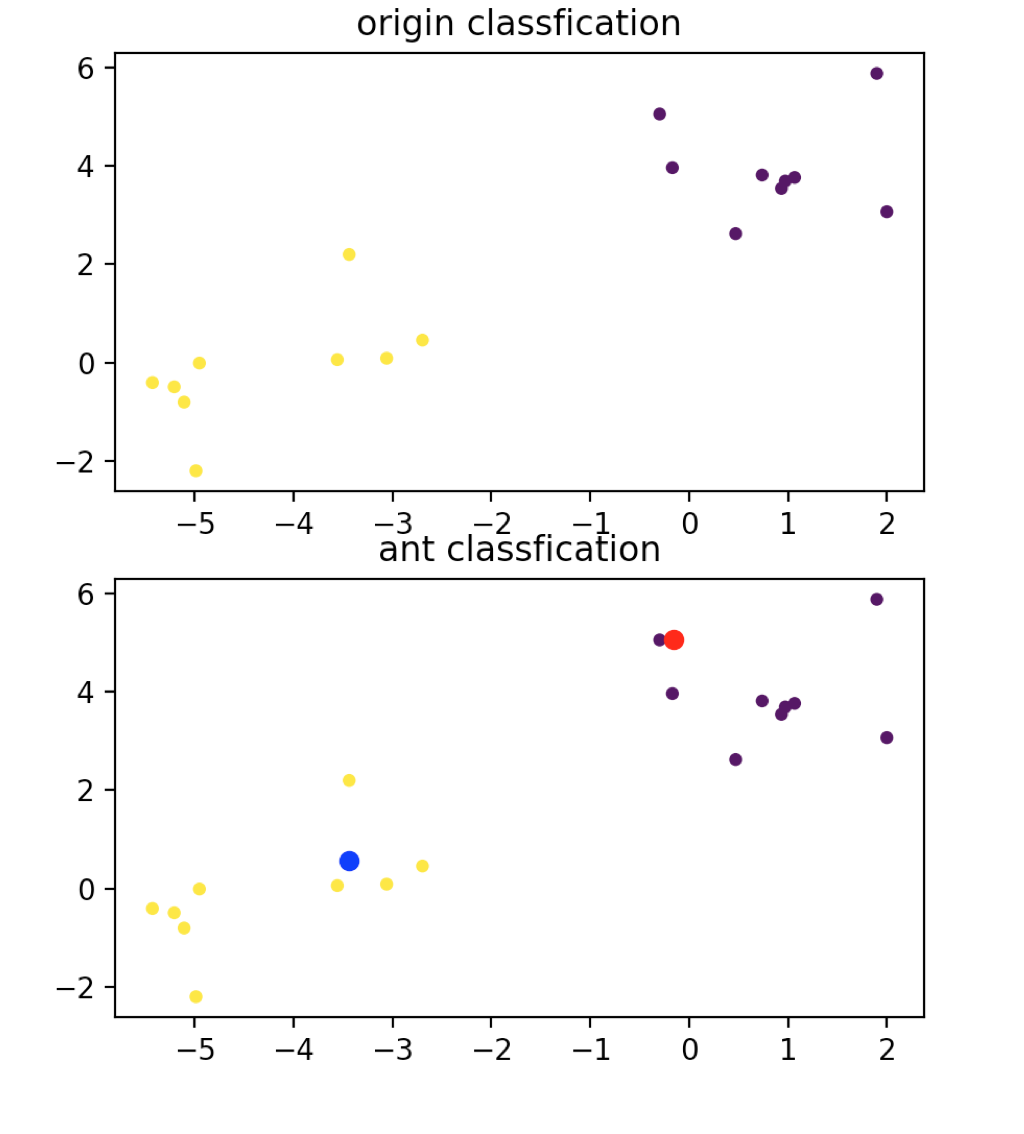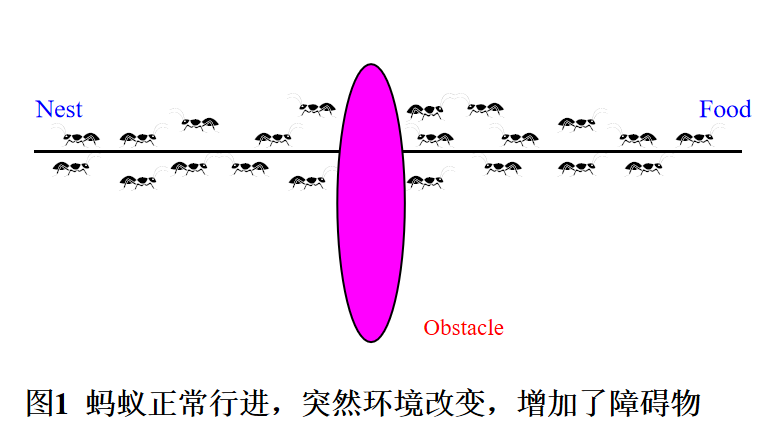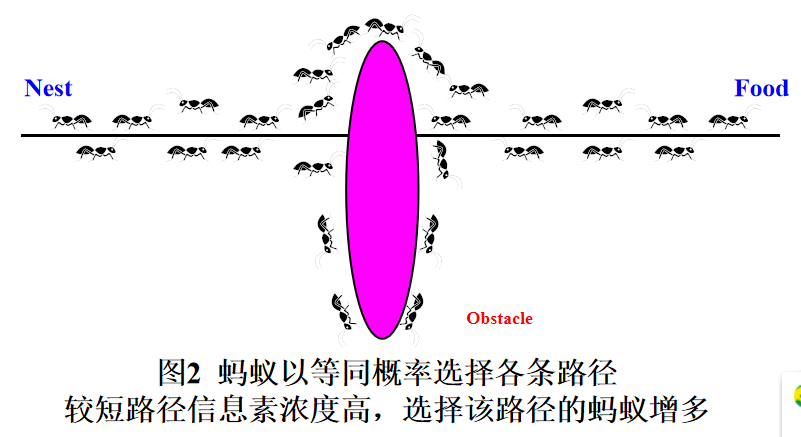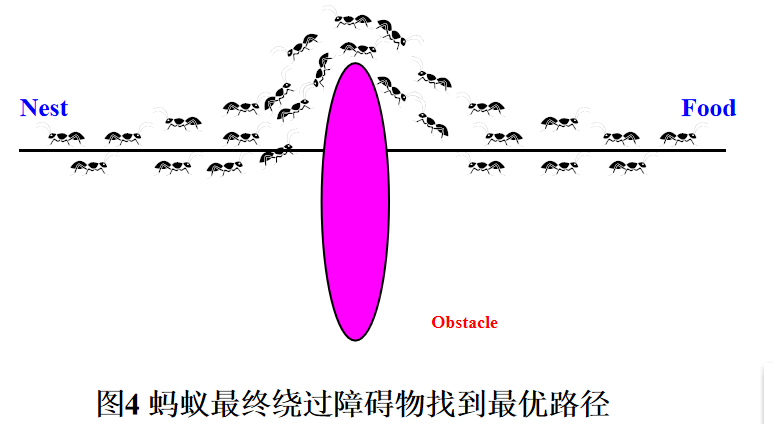• 蚁群算法是经典的算法、本程序主要用于蚁群算法进行聚类分析，处理数据
• 转载一个大哥的蚁群算法聚类优化，不是本人原创，希望通过审批，造福大家，该算法将扩大最优解蚁群算法聚类
• 蚁群算法聚类分析 摘要 蚁群算法是今年来才提出的一种基于种群寻优的启发式搜索算法由意大利学者M.Dorigo等于1991年首先提出该算法受到自然界中真实蚁群集体行为的启发利用真实蚁群通过个体间的信息传递搜索从蚁穴到...
•matlab
• 蚁群聚类算法的matlab实现，有说明和详尽的报告。
• 利用蚁群算法得到模糊聚类的初始值，再利用FCM对样本数据进行分类。
• 【2】《蚁群聚类算法综述》 1 算法概述 聚类数已知的算法流程 初始化蚁群参数，如蚂蚁数量、聚类数量等；每只蚂蚁对应一个解集： 样品号 1 2 … N 蚂蚁SiS_iSi​ 类别1 类别2 … 类别1 上述表表示蚂蚁...

【参考资料】
【1】《模式识别与智能计算的MATLAB技术实现》
【2】《蚁群聚类算法综述》

1 算法概述

聚类数已知的算法流程
1. 初始化蚁群参数，如蚂蚁数量、聚类数量等；每只蚂蚁对应一个解集：
样品号12N
蚂蚁 S i S_i 类别1类别2类别1

上述表表示蚂蚁 S i S_i 把N个样本分归属到分类中。

1. 构建信息素矩阵
样本\类别类别1类别2类别3类别4
样本10.10.10.10.1
样本20.10.10.10.1
样本30.10.10.10.1
0.10.10.10.1
样本N0.10.10.10.1

上述信息素 τ i j \tau_{ij} 表示把第i个样本归类到第j个类别时的信息素

1. 构建目标函数

假设: N个样品、M个模式分类 ∣ S j , j = 1 , 2 , . . . , M ∣ |S_j,j=1,2,..., M| ,每个样品有n个特征。
目标: 将每个样品到聚类中心的距离和达到最小

m i n J ( w , c ) = ∑ j = 1 m ∑ i = 1 N ∑ p = 1 n w i j ∣ ∣ x i p − c j p ∣ ∣ 2 minJ(w, c)=\sum\limits_{j=1}^{m}\sum\limits_{i=1}^{N}\sum\limits_{p=1}^{n} w_{ij}||x_{ip} - c_{jp}||^2

c j p = ∑ i = 1 N w i j x i p ∑ i = 1 N w i j c_{jp}=\dfrac{\sum\limits_{i=1}^{N}w_{ij}x_{ip}}{\sum\limits_{i=1}^{N}w_{ij}}

w i j = { 1 , N i ∈ S j 0 , N i ∉ S j w_{ij} = \begin{cases} 1, &amp; N_i \in S_j \\ 0, &amp; N_i \notin S_j \end{cases} 样本属于该类则为1，否则为0

其中 x i p x_{ip} 为第i个样本的p属性、 c j p c_{jp} 为第j个分类的p属性

1. 更新蚁群

每一只蚂蚁在对自己解集中样本归属判断时采用两种策略（随机选择）：

1. 根据当前时刻信息素表，选择信息素高的；（直接基于既有知识）
2. 按照当前信息素的概率，即信息素高的类别有更大的可能性被选择；（也是基于既有知识，但非直接，而是一定程度上的随机选择）

4.1 参考第一步每只蚂蚁所具备的解集；根据目标函数公式计算每只蚂蚁的目标值；
4.2 根据目标值将蚂蚁进行排序；
4.3 取最优的L只蚂蚁进行“局部搜索”,遍历这L只蚂蚁:

4.3.1 随机选择其中第i个样本，重新计算目标函数，确定一个新的分类;
4.3.2 当这只蚂蚁的若干个样本被重新分类后，再计算一次该蚂蚁的总目标函数，若解更优；则替换原解;
4.3.3 遍历L只蚂蚁后，前L只蚂蚁中具备最优解的作为当前全局最优解;

1. 更新信息素表
τ i j ( t + 1 ) = ( 1 − ρ ) τ i j ( t ) + ∑ s = 1 l Δ τ i j s \tau_{ij}(t+1)=(1-\rho)\tau_{ij}(t) + \sum\limits_{s=1}^{l}\Delta \tau_{ij}^s
其中若蚂蚁s中的样品i属于分类j，则 Δ τ i j s = Q J \Delta \tau_{ij}^s=\dfrac{Q}{J} ，否则为0。这里Q是一个超参数；J是蚂蚁s的目标函数值。

2. 多次迭代后达到全局最优解

2 算法实现

实际在实现过程中感觉蚁群算法还是非常收到超参数影响。包括信息素的挥发参数，以及超参数Q等等，而且效果差距非常大。当前代码中的参数是一个具备比较好效果的设置。

# -*- coding: utf-8 -*-
import numpy  as np
import sklearn.datasets as ds
import matplotlib.pyplot as plt
import random
import math
import operator

SAMPLE_NUM  = 18    #样本数量
FEATURE_NUM = 2     #每个样本的特征数量
CLASS_NUM   = 2     #分类数量
ANT_NUM     = 200    #蚂蚁数量

"""
初始化测试样本，sample为样本，target_classify为目标分类结果用于对比算法效果
"""
sample, target_classify = ds.make_blobs(SAMPLE_NUM, n_features=FEATURE_NUM, centers=CLASS_NUM, random_state=3)

"""
信息素矩阵
"""
tao_array =  [[random.random() for col in range(FEATURE_NUM)] for row in range(SAMPLE_NUM)]

"""
蚁群解集
"""
ant_array = [[0 for col in range(SAMPLE_NUM)] for row in range(ANT_NUM)]

t_ant_array = [[0 for col in range(SAMPLE_NUM)] for row in range(ANT_NUM)] #存储局部搜索时的临时解

"""
聚类中心点
"""
center_array = [[0 for col in range(FEATURE_NUM)] for row in range(CLASS_NUM)]

"""
当前轮次蚂蚁的目标函数值，前者是蚂蚁编号、后者是目标函数值
"""
ant_target = [(0, 0) for col in range(ANT_NUM)]

change_q   = 0.3       #更新蚁群时的转换规则参数，表示何种比例直接根据信息素矩阵进行更新
L          = 2         #局部搜索的蚂蚁数量
change_jp  = 0.03      #局部搜索时该样本是否变动
change_rho = 0.02      #挥发参数
Q          = 0.1      #信息素浓度参数

def _init_test_data():

"""
初始化蚁群解集，随机确认每只蚂蚁下每个样本的分类为1或者0
"""
for i in range(0, ANT_NUM):
for j in range(0, SAMPLE_NUM):

tmp = random.randint(0, FEATURE_NUM - 1)

ant_array[i][j] = tmp

"""
将前两个样本作为聚类中心点的初始值
"""
for i in range(0, CLASS_NUM):

center_array[i] = sample[random.randint(0, SAMPLE_NUM-1)]
center_array[i] = sample[random.randint(0, SAMPLE_NUM-1)]

def _get_best_class_by_tao_value(sampleid):

max_value = np.max(tao_array[sampleid])

for i in range(0, CLASS_NUM):

if max_value == tao_array[sampleid][i]:

return i

def random_pick(some_list, probabilities):
x = random.uniform(0,1)
cumulative_probability = 0.0
for item, item_probability in zip(some_list, probabilities):
cumulative_probability += item_probability
if x < cumulative_probability:break
return item

def _get_best_class_by_tao_probablity(sampleid):

tarray = np.array(tao_array[sampleid])

parray = tarray/np.sum(tarray)

return random_pick([0,1], parray)

def _update_ant():

"""
更新蚁群步骤
"""

#产生一个随机数矩阵
r = np.random.random((ANT_NUM, SAMPLE_NUM))

for i in range(0, ANT_NUM):
for j in range(0, SAMPLE_NUM):

if r[i][j] > change_q:

tmp_index = _get_best_class_by_tao_value(j)

#选择该样本中信息素最高的做为分类
ant_array[i][j] = tmp_index

else:
#计算概率值，根据概率的大小来确定一个选项
tmp_index = _get_best_class_by_tao_probablity(j)

ant_array[i][j] = tmp_index

#print(ant_array[i])
#1. 确定一个新的聚类中心
f_value_feature_0 = 0
f_value_feature_1 = 0

for i in range(0, CLASS_NUM):

f_num   = 0

for j in range(0, ANT_NUM):

for k in range(0, SAMPLE_NUM):

if ant_array[j][k] == 0:

f_num   += 1
f_value_feature_0 += sample[k] #特征1

else:

f_num   += 1
f_value_feature_1 += sample[k] #特征2

if i == 0:
center_array[i] = f_value_feature_0/f_num
else:
center_array[i] = f_value_feature_1/f_num

#print(center_array[i], f_num)

def _judge_sample(sampleid):

"""
计算与当前聚类点的举例，判断该sample应所属的归类
"""
target_value_0 = 0
target_value_1 = 0

f1 = math.pow((sample[sampleid] - center_array),2)
f2 = math.pow((sample[sampleid] - center_array),2)
target_value_0 = math.sqrt(f1 + f2)

f1 = math.pow((sample[sampleid] - center_array),2)
f2 = math.pow((sample[sampleid] - center_array),2)
target_value_1 = math.sqrt(f1 + f2)

if target_value_0 > target_value_1:
return 1
else:
return 0

def _local_search():

"""
局部搜索逻辑
"""

#2. 根据新的聚类中心计算每个蚂蚁的目标函数

for i in range(0, ANT_NUM):

target_value = 0

for j in range(0, SAMPLE_NUM):

if ant_array[i][j] == 0:

#与分类0的聚类点计算距离
f1 = math.pow((sample[j] - center_array),2)
f2 = math.pow((sample[j] - center_array),2)
target_value += math.sqrt(f1 + f2)

else:
#与分类1的聚类点计算距离
f1 = math.pow((sample[j] - center_array),2)
f2 = math.pow((sample[j] - center_array),2)
target_value += math.sqrt(f1 + f2)

#保存蚂蚁i当前的目标函数
ant_target[i] = (i, target_value)

#3. 对全部蚂蚁的目标进行排序，选择最优的L只蚂蚁

ant_target.sort(key= operator.itemgetter(1)) #对ant进行排序

#4. 对这L只蚂蚁进行解的优化
for i in range(0, L):

ant_id = ant_target[i]

target_value = 0

for j in range(0, SAMPLE_NUM):

#对于该蚂蚁解集中的每一个样本
if random.random() < change_jp:

#将该样本调整到与当前某个聚类点最近的位置
t_ant_array[ant_id][j] = _judge_sample(j)

#判断是否保留这个临时解
for j in range(0, SAMPLE_NUM):

if t_ant_array[ant_id][j] == 0:

#与分类0的聚类点计算距离
f1 = math.pow((sample[j] - center_array),2)
f2 = math.pow((sample[j] - center_array),2)
target_value += math.sqrt(f1 + f2)

else:
#与分类1的聚类点计算距离
f1 = math.pow((sample[j] - center_array),2)
f2 = math.pow((sample[j] - center_array),2)
target_value += math.sqrt(f1 + f2)

if target_value < ant_target[i]:

#更新最优解
ant_array[ant_id] = t_ant_array[ant_id]

def _update_tau_array():

"""
更新信息素表
"""
for i in range(0, SAMPLE_NUM):

for j in range(0, CLASS_NUM):

tmp = tao_array[i][j] #当前的信息素

tmp = (1 - change_rho) * tmp #处理信息素挥发

J = 0

#处理信息素浓度增加
for k in range(0, ANT_NUM):

if ant_array[k][i] == j:

f1 = math.pow((sample[i] - center_array[j]),2)
f2 = math.pow((sample[i] - center_array[j]),2)
J += math.sqrt(f1 + f2)

if J != 0:
tmp += Q/J

#print(tmp, Q/J)

tao_array[i][j] = tmp

#print(np.var(tao_array))

"""
说明：

简单蚁群算法解决聚类问题，参考笔记《蚁群算法-聚类算法》

作者：fredric

日期：2018-12-21

"""
if __name__ == "__main__":

_init_test_data();

for i in range(0, 100):

print("iterate No. {} target {}".format(i, ant_target))

_update_ant()

_local_search()

_update_tau_array()

#画出分类
pre = ant_array[ant_target]

plt.figure(figsize=(5, 6), facecolor='w')
plt.subplot(211)
plt.title('origin classfication')
plt.scatter(sample[:, 0], sample[:, 1], c=target_classify, s=20, edgecolors='none')

plt.subplot(212)
plt.title('ant classfication')
plt.scatter(sample[:, 0], sample[:, 1], c=pre, s=20, edgecolors='none')

plt.plot(center_array, center_array,'ro')
plt.plot(center_array, center_array,'bo')

plt.show()展开全文机器学习 蚁群算法 聚类
• 1 蚁群算法的提出 蚁群算法(ant colony optimization, ACO)，又称蚂蚁算法，是一种用来寻找优化路径的机率型算法。它由Marco Dorigo于1992年在他的博士论文中提出，其灵感来源于蚂蚁在寻找食物过程中发现路径的行为...

一、获取代码方式

获取代码方式1：
完整代码已上传我的资源：【数据聚类】基于matlab蚁群算法聚类设计【含Matlab源码 202期】

获取代码方式2：
通过紫极神光博客主页开通CSDN会员，凭支付凭证，私信博主，可获得此代码。

获取代码方式3：
通过订阅紫极神光博客付费专栏，凭支付凭证，私信博主，可获得此代码。

备注：开通CSDN会员，仅只能免费获得1份代码（有效期为开通日起，三天内有效）；
订阅紫极神光博客付费专栏，可免费获得2份代码（有效期为订阅日起，三天内有效）；

二、蚁群算法简介

1 蚁群算法的提出
蚁群算法(ant colony optimization, ACO)，又称蚂蚁算法，是一种用来寻找优化路径的机率型算法。它由Marco Dorigo于1992年在他的博士论文中提出，其灵感来源于蚂蚁在寻找食物过程中发现路径的行为。遗传算法在模式识别、神经网络、机器学习、工业优化控制、自适应控制、生物科学、社会科学等方面都得到应用。

2 算法的基本原理三、部分源代码

clc;
clf;
clear;
% X = 测试样本矩阵;
X=[
2232.43	3077.87	1298.87;
1580.1	1752.07	2463.04;
1962.4	1594.97	1835.95;
1495.18	1957.44	3498.02;
1125.17	1594.39	2937.73;
24.22	3447.31	2145.01;
1269.07	1910.72	2701.97;
1802.07	1725.81	1966.35;
1817.36	1927.4	2328.79;
1860.45	1782.88	1875.13;
1237.91	2055.13	3405.09;
688.94	2104.72	3198.51;
1675.65	1747.23	1580.39;
1806.02	1810.19	2191.12;
74.56	3288.02	2433.87;
307.35	3363.84	2021.61;
1988.27	1657.51	2069.2;
2173.92	2608.55	1803.57;
372.16	3077.44	2163.46;
576.6	2140.98	3320;
1724.13	1704.49	1798.75;
2501.21	2652.65	984.56;
1656.94	1913.34	2459.07;
362.51	3150.03	2472;
565.74	2284.97	3024.58;
1978.06	1536.13	2375.64;
1661.06	1552.4	2005.05;
790.29	2419.98	3051.16;
1557.27	1746.27	1879.13;
2793.36	3009.26	1073.55;
1766.08	1803.14	1895.18;
1207.88	1600.62	3123.07;
245.75	3373.67	2248.45;
2785.36	3052.81	1035.65;
315.42	3088.29	2187.12;
1243.28	2451.72	3111.99;
829.84	1555.91	3139.21;
1347.07	2364.31	3096.88;
1926.98	1507.34	1626.47;
1808.57	1608.78	1565.95;
1124.1	1840.98	2819.41;
2661	3302.39	1710.32;
1805.55	1899.09	2400.6;
1130.18	1902.42	2753.7;
1355.19	1566.16	2927.81;
1651.14	1774.03	1725.56;
2110.63	3308.04	702.06;
2788.11	3395.23	1684.45;
1807.61	1680.56	2356.65;
1363.58	1729.44	2749.55;
1992.42	1526.9	1581.42;
]
[N,n]=size(X);      % N =测试样本数;n =测试样本的属性数;
K = 4;              % K = 组数;
R = 100;            % R = 蚂蚁数;
% 初始化
c = 10^-2;
tau = ones(N,K) * c;    %信息素矩阵，初始值为0.01的N*K矩阵（样本数*聚类数）
rho = 0.1;              % 蒸发率
best_solution_function_value = inf; % 最佳路径度量值（初值为无穷大，该值越小聚类效果越好）
tic
t = 1;
%=======程序终止条件（下列两个终止条件任选其一）======
% while ((t<=t_max))                             %达到最大迭代次数而终止
% while ((best_solution_function_value>=19727))  %达到一定的聚类效果而终止
while ((best_solution_function_value>=19727))
%=========================

四、运行结果四、matlab版本及参考文献

1 matlab版本
2014a

2 参考文献
 包子阳,余继周,杨杉.智能优化算法及其MATLAB实例（第2版）[M].电子工业出版社，2016.
张岩,吴水根.MATLAB优化算法源代码[M].清华大学出版社，2017.

展开全文matlab 算法
• 针对社交网络中社交关系的有向性与多样性，提出了一种基于图聚类蚁群算法的社交网络聚类算法。首先，在网络覆盖率的约束下为社交网络建立有向、非全连接的二维图模型；然后，采用K-medoids算法搜索用户分组的中心...
• 蚁群聚类算法及其源码, 可用于完成毕业设计作参考，其中就涉及到蚁群算法聚类分析，自己编写的程序实现效果有待提高。
• 该算法，解决了不收敛的问题，聚类效果非常好（效果图如附件图片所示）。改进的蚁群算法是基于遗传算法的改进，在基本遗传算法的基础之上，加入了变异因子，产生变异，从而更快的收敛。
• 基本蚁群聚类算法及其改进算法带Matlab源代码-蚁群算法.ppt 基本蚁群聚类算法及其改进算法[带Matlab源代码]  该算法，解决了不收敛的问题，聚类效果非常好（效果图如附件图片所示）。改进的蚁群算法是基于遗传...matlab
• 给出了一种改进的蚁群算法聚类程序，比基本蚁群聚类效果好
• 将核蚁群化学聚类算法用于三个标准数据集合，计算结果表明与蚁群化学聚类算法相比，核蚁群化学聚类算法聚类效果提升显著。将核蚁群化学聚类算法、核自组织神经网络映射算法和基于多项式核的结构化有向树数据聚类算法...径向基核函数
• 蚁群算法matlab代码基于蚁群的聚类 MATLAB 中基于蚁群的聚类 描述 MATLAB 代码 ACOmain.m 是一个简单的 ACO 算法实现，使用 4 个高斯分布的合成数据集进行编码。 运行时的代码提供了处理数据的蚁群的漂亮可视化。 ...
• 基于蚁群算法的动态模糊聚类分析，黄红星，，本文提出了一种基于蚁群算法的动态模糊聚类方法。算法将蚁群算法与模糊C均值聚类有机的结合，实现了基于改进的目标函数聚类分析��
• 模式识别与人工智能：基于MATLAB 第9章　蚁群算法聚类设计 蚁群优化算法是由意大利学者M. Dorigo等人提出的一种新型的解决组合优化问题的模拟进化算法。该算法不仅能够实现智能搜索、全局优化，而且具有稳健性、正...
• 基于蚁群模糊聚类算法的图像边缘检测，黄红星， 苗 京，本文提出了一种基于蚁群动态模糊聚类算法的图像边缘检测。算法将蚁群算法与模糊C均值聚类有机的结合，实现了基于改进的目标函数��
• 针对模糊C-均值算法对初始值的依赖,容易陷入局部最优值的缺点,本文提出将量子蚁群算法与FCM聚类算法结合,首先利用量子蚁群算法的全局性和鲁棒性以及快速收敛的优点确定图像的初始聚类中心和聚类个数,再将所得结果...
• 蚁群聚类算法

2018-01-25 17:27:08
蚁群聚类算法，已验证，可行！可以分析样品分类问题，程序简单清晰有效，可供matlab运行使用，。蚁群聚类算法
• 基于遗传算法的改进，在基本遗传算法的基础之上，加入了变异因子，产生变异，从而更快的收敛。
• 蚁群聚类算法及其源码matlab-蚁群聚类算法及其源码[matlab].rar 第一次发帖子就不要钱了哈哈 程序代码： 上周忙着看公钥算法，基础要补的太多，看得晕晕的～～周末去了一趟河北，和博士一起去的，看到久违...matlab
• 蚁群算法matlab

千次阅读 多人点赞 2018-09-12 16:49:55
（一）蚁群算法的由来 蚁群算法最早是由Marco Dorigo等人在1991年提出，他们在研究新型算法的过程中，发现蚁群在寻找食物时，通过分泌一种称为信息素的生物激素交流觅食信息从而能快速的找到目标，据此提出了基于...

（一）蚁群算法的由来

蚁群算法最早是由Marco Dorigo等人在1991年提出，他们在研究新型算法的过程中，发现蚁群在寻找食物时，通过分泌一种称为信息素的生物激素交流觅食信息从而能快速的找到目标，据此提出了基于信息正反馈原理的蚁群算法。

蚁群算法的基本思想来源于自然界蚂蚁觅食的最短路径原理，根据昆虫科学家的观察，发现自然界的蚂蚁虽然视觉不发达，但它们可以在没有任何提示的情况下找到从食物源到巢穴的最短路径，并在周围环境发生变化后，自适应地搜索新的最佳路径。

蚂蚁在寻找食物源的时候，能在其走过的路径上释放一种叫信息素的激素，使一定范围内的其他蚂蚁能够察觉到。当一些路径上通过的蚂蚁越来越多时，信息素也就越来越多，蚂蚁们选择这条路径的概率也就越高，结果导致这条路径上的信息素又增多，蚂蚁走这条路的概率又增加，生生不息。这种选择过程被称为蚂蚁的自催化行为。对于单个蚂蚁来说，它并没有要寻找最短路径，只是根据概率选择；对于整个蚁群系统来说，它们却达到了寻找到最优路径的客观上的效果。这就是群体智能。

（二）蚁群算法能做什么

蚁群算法根据模拟蚂蚁寻找食物的最短路径行为来设计的仿生算法，因此一般而言，蚁群算法用来解决最短路径问题，并真的在旅行商问题（TSP，一个寻找最短路径的问题）上取得了比较好的成效。目前，也已渐渐应用到其他领域中去，在图着色问题、车辆调度问题、集成电路设计、通讯网络、数据聚类分析等方面都有所应用。

（三）蚁群算法的流程步骤
这里以TSP问题为例，算法设计的流程如下：

• 步骤1：对相关参数进行初始化，包括蚁群规模、信息素因子、启发函数因子、信息素挥发因子、信息素常数、最大迭代次数等，以及将数据读入程序，并进行预处理：比如将城市的坐标信息转换为城市间的距离矩阵。

• 步骤2：随机将蚂蚁放于不同出发点，对每个蚂蚁计算其下个访问城市，直到有蚂蚁访问完所有城市。

• 步骤3：计算各蚂蚁经过的路径长度Lk，记录当前迭代次数最优解，同时对路径上的信息素浓度进行更新。

• 步骤4：判断是否达到最大迭代次数，若否，返回步骤2；是，结束程序。

• 步骤5：输出结果，并根据需要输出寻优过程中的相关指标，如运行时间、收敛迭代次数等。

要用到的符号说明：

m：整个蚂蚁群体中蚂蚁数量；
n：城市的数量；
dij：城市i与城市j的距离
βij(t)：t时刻城市i和城市j连接路径上的信息素；
pkij(t)：t时刻蚂蚁k从城市i转移到城市j的概率；

初始时刻蚂蚁被放在不同的城市，且各城市路径上的信息素浓度为0。

由于蚁群算法涉及到的参数蛮多的，且这些参数的选择对程序又都有一定的影响，所以选择合适的参数组合很重要。蚁群算法有个特点就是在寻优的过程中，带有一定的随机性，这种随机性主要体现在出发点的选择上。蚁群算法正是通过这个初始点的选择将全局寻优慢慢转化为局部寻优的。参数设定的关键就在于在“全局”和“局部”之间建立一个平衡点。

（四）蚁群算法的关键参数

在蚁群算法的发展中，关键参数的设定有一定的准则，一般来讲遵循以下几条：

尽可能在全局上搜索最优解，保证解的最优性；

算法尽快收敛，以节省寻优时间；

尽量反应客观存在的规律，以保证这类仿生算法的真实性。

蚂蚁数量：
设M表示城市数量，m表示蚂蚁数量。m的数量很重要，因为m过大时，会导致搜索过的路径上信息素变化趋于平均，这样就不好找出好的路径了；m过小时，易使未被搜索到的路径信息素减小到0，这样可能会出现早熟，没找到全局最优解。一般上，在时间等资源条件紧迫的情况下，蚂蚁数设定为城市数的1.5倍较稳妥。

信息素因子：
信息素因子反映了蚂蚁在移动过程中所积累的信息量在指导蚁群搜索中的相对重要程度，其值过大，蚂蚁选择以前走过的路径概率大，搜索随机性减弱；值过小，等同于贪婪算法，使搜索过早陷入局部最优。实验发现，信息素因子选择[1,4]区间，性能较好。

启发函数因子：
启发函数因子反映了启发式信息在指导蚁群搜索过程中的相对重要程度，其大小反映的是蚁群寻优过程中先验性和确定性因素的作用强度。过大时，虽然收敛速度会加快，但容易陷入局部最优；过小时，容易陷入随机搜索，找不到最优解。实验研究发现，当启发函数因子为[3,4.5]时，综合求解性能较好。

信息素挥发因子：
信息素挥发因子表示信息素的消失水平，它的大小直接关系到蚁群算法的全局搜索能力和收敛速度。实验发现，当属于[0.2，0.5]时,综合性能较好。

信息素常数：
这个参数为信息素强度，表示蚂蚁循环一周时释放在路径上的信息素总量，其作用是为了充分利用有向图上的全局信息反馈量，使算法在正反馈机制作用下以合理的演化速度搜索到全局最优解。值越大，蚂蚁在已遍历路径上的信息素积累越快，有助于快速收敛。实验发现，当值属于[10,1000]时，综合性能较好。

最大迭代次数：
最大迭代次数值过小，可能导致算法还没收敛就已结束；过大则会导致资源浪费。一般最大迭代次数可以取100到500次。一般来讲，建议先取200，然后根据执行程序查看算法收敛的轨迹来修改取值。

组合参数设计策略：
通常可以按照以下策略来进行参数组合设定：

1. 确定蚂蚁数目，蚂蚁数目与城市规模之比约为1.5；
2. 参数粗调，即调整取值范围较大的α,β及Q；
3. 参数微调，即调整取值范围较小的ρ
%设置初始化参数

C=[1,2;70,90;80,60;10,100;800,200;800,100;90,80;200,600;230,4;500,90];

NC_max=100;
m=18;
Alpha=1;
Beta=5;
Rho=0.5;
Q=1;
%%-------------------------------------------------------------------------
%% 主要符号说明
%% C n个城市的坐标，n×2的矩阵
%% NC_max 最大迭代次数
%% m 蚂蚁个数
%% Alpha 表征信息素重要程度的参数
%% Beta 表征启发式因子重要程度的参数
%% Rho 信息素蒸发系数
%% Q 信息素增加强度系数
%% R_best 各代最佳路线
%% L_best 各代最佳路线的长度
%%=========================================================================

%%第一步：变量初始化
n=size(C,1);%n表示问题的规模（城市个数）
D=zeros(n,n);%D表示完全图的赋权邻接矩阵
for i=1:n
for j=1:n
if i~=j
D(i,j)=((C(i,1)-C(j,1))^2+(C(i,2)-C(j,2))^2)^0.5;
else
D(i,j)=eps;      %i=j时不计算，应该为0，但后面的启发因子要取倒数，用eps（浮点相对精度）表示
end
D(j,i)=D(i,j);   %对称矩阵
end
end
%{

1.C就是城市坐标

x         y

城市1    0     120

城市2   120     0

城市n   100    230

2.运行后这里D变成了一个n*n的矩阵，每个元素代表两个城市之间的距离，比如当城市数目为3时：

D=      城市1    城市2    城市3

城市1   0        120      100

城市2  120       0        230

城市3  100    230         0

这里D是个对角线为0的对称矩阵，因为城市1,2间距离等于城市2,1的距离，城市n与n距离设置为0

%}

Eta=1./D;          %Eta为启发因子矩阵，这里设为距离的倒数
Tau=ones(n,n);     %Tau为信息素矩阵
Tabu=zeros(m,n);   %存储并记录路径的生成，禁忌表
NC=1;               %迭代计数器，记录迭代次数
R_best=zeros(NC_max,n);       %各代最佳路线
L_best=inf.*ones(NC_max,1);   %各代最佳路线的长度
L_ave=zeros(NC_max,1);        %各代路线的平均长度

while NC<=NC_max        %停止条件之一：达到最大迭代次数，停止
%%第二步：将m只蚂蚁放到n个城市上
Randpos=[];   %随机存取
for i=1:(ceil(m/n))
Randpos=[Randpos,randperm(n)];
end
Tabu(:,1)=(Randpos(1,1:m))';    %此句不太理解？

%{

1.ceil(m/n)

假如有 10只蚂蚁，3个城市，ceil(m/n)=ceil（10/3=4，需要安排四次，才能把这十只蚂蚁全部放到到三个城市，

每次都在行向量Randpos加入如新的元素，randperm(3)表示就是1 3 2，或者3 1 2这种随机组合，4次循环之后，

那么Randpos =

2     3     1     1     2     3     3     1     2     3     2     1

2.Tabu(:,1)=(Randpos(1,1:m))'

总共m蚂蚁，只这里m为10，Tabu(:,1)表示Tabu第一行就是初始10只蚂蚁被随机分到所三个城市中的一个

Tabu =

2
3
1
1
2
3
3
1
2
3

这里只取m=10个数，因为Tabu第一列表示m只蚂蚁初始的时候随机被分在的城市，比如第一个2代表，第一只蚂蚁

最开始放在了城市2，以此类推

%}

%%第三步：m只蚂蚁按概率函数选择下一座城市，完成各自的周游
for j=2:n     %所在城市不计算
for i=1:m
visited=Tabu(i,1:(j-1)); %记录已访问的城市，避免重复访问
J=zeros(1,(n-j+1));       %待访问的城市
P=J;                      %待访问城市的选择概率分布
Jc=1;                       %访问的城市个数
for k=1:n
if length(find(visited==k))==0   %开始时置0
J(Jc)=k;                           %这时记录没有访问的城市到J中
Jc=Jc+1;                         %访问的城市个数自加1
end
end

%{

1.visited=Tabu(i,1:(j-1));    向量visited记录已访问的城市，比如第一次Tabu中第一行第一个的城市2

2.J=zeros(1,(n-j+1))           向量J记录待访问的城市，已结访问城市2，还没访问13城市放入J向量中

3.if length(find(visited==k))==0
判断语句，find()语句找到visited中等于k的元素在数组visited中的位置，例如数组a=[1 2 3 4 5 2],

find(a==2)=[2,6]，find(a==6)=[],则
length(find(a==6))=0
length()==0判断length()是否为零
如果为零就是visited中没有k元素，即没有访问过k城市。
这时记录没有访问的城市到J中。

%}

%下面计算待选城市的概率分布
for k=1:length(J)
P(k)=(Tau(visited(end),J(k))^Alpha)*(Eta(visited(end),J(k))^Beta);

end
P=P/(sum(P));
%按概率原则选取下一个城市
Pcum=cumsum(P);     %cumsum，元素累加即求和
Select=find(Pcum>=rand); %若计算的概率大于原来的就选择这条路线%要选择其中总概率大于等于某一个随机数，找到大于等于这个随机数的城市的在J中的位置
to_visit=J(Select(1));  %提取这些城市的编号到to_visit中
Tabu(i,j)=to_visit;
end
end

%{

1. %visited(end)表示蚂蚁现在所在城市编号，J(k)表示下一步要访问的城市编号

2.P=P/(sum(P));把各个路径概率统一到和为1

3.Pcum=cumsum(P);   cumsum，元素累加即求和，比如P=[0.1 0.5 0.4]，cumsum(P)=  [0.1000    0.6000    1.0000]

有一点要特别说明，用到cumsum(P)，蚂蚁要选择的下一个城市不是按最大概率，就是要用到轮盘法则，不然影响全局收缩能力，

所以用到累积函数，Pcum=cumsum(P)

4.Select=find(Pcum>=rand); to_visit=J(Select(1))

轮盘法则，Select(1)1保证可以选到最大概率的城市，具体自己可以用matlab试一下：

p=[0.1 0.6 0.3]    中间那个城市概率最大

此时Pcum=[0.1  0.7  1],   Select =[2   3];  Select(1)=2,中间那个城市概率最大

%}

if NC>=2
Tabu(1,:)=R_best(NC-1,:);
end

%%第四步：记录本次迭代最佳路线
L=zeros(m,1);     %开始距离为0，m*1的列向量
for i=1:m
R=Tabu(i,:);
for j=1:(n-1)
L(i)=L(i)+D(R(j),R(j+1));    %原距离加上第j个城市到第j+1个城市的距离
end
L(i)=L(i)+D(R(1),R(n));      %一轮下来后走过的距离，加上第一个和最后一个城市的距离
end

%{

1.L=zeros(m,1)   记录本次迭代最佳路线的长度，每个蚂蚁都有自己走过的长度记录在向量L中

%}

L_best(NC)=min(L);           %最佳距离取最小
pos=find(L==L_best(NC));   %找到路径最短的那条蚂蚁所在的行编号
R_best(NC,:)=Tabu(pos(1),:); %此轮迭代后的最佳路线
L_ave(NC)=mean(L);           %此轮迭代后的平均距离
NC=NC+1                      %迭代继续

%{

1.R_best(NC,:)=Tabu(pos(1),:)：找到路径最短的那条蚂蚁所在的城市先后顺序，pos(1)1表示万一有长度一样的两条蚂蚁，那就选第一个

%}

%%第五步：更新信息素
Delta_Tau=zeros(n,n);        %开始时信息素为n*n的0矩阵
for i=1:m
for j=1:(n-1)
Delta_Tau(Tabu(i,j),Tabu(i,j+1))=Delta_Tau(Tabu(i,j),Tabu(i,j+1))+Q/L(i);
%此次循环在路径（i，j）上的信息素增量
end
Delta_Tau(Tabu(i,n),Tabu(i,1))=Delta_Tau(Tabu(i,n),Tabu(i,1))+Q/L(i);    %加上第一个到最后一个城市的信息素增量
%此次循环在整个路径上的信息素增量
end
Tau=(1-Rho).*Tau+Delta_Tau; %考虑信息素挥发，更新后的信息素
%%第六步：禁忌表清零
Tabu=zeros(m,n);             %%直到最大迭代次数
end

%{

1.R_best(NC,:)=Tabu(pos(1),:)：找到路径最短的那条蚂蚁所在的城市先后顺序，pos(1)1表示万一有长度一样的两条蚂蚁，那就选第一个

%}

%%第七步：输出结果
Pos=find(L_best==min(L_best)); %找到最佳路径（非0为真）
Shortest_Route=R_best(Pos(1),:) %最大迭代次数后最佳路径
Shortest_Length=L_best(Pos(1)) %最大迭代次数后最短距离
subplot(1,2,1)                  %绘制第一个子图形

N=length(R);
scatter(C(:,1),C(:,2));
hold on
plot([C(R(1),1),C(R(N),1)],[C(R(1),2),C(R(N),2)],'r')
hold on
for ii=2:N
plot([C(R(ii-1),1),C(R(ii),1)],[C(R(ii-1),2),C(R(ii),2)],'r')
hold on
end

title('旅行商问题优化结果 ')

subplot(1,2,2)                  %绘制第二个子图形
plot(L_best)
hold on                         %保持图形
plot(L_ave,'r')
title('平均距离和最短距离')     %标题

%{

这部分没什么太大问题，多看几遍就好

%}

展开全文• 蚁群算法ACO python实现 1.0版本 sugarMei 2020 5-27 """ # 初始化参数 ''' alpha:信息素影响的强度大小 beta:可见度影响的强度大小 rho:信息素挥发因子 q:常数 用于计算每次一次遍历后的信息素强度变化程度 eta:...
import tkinter
from functools import reduce
import time

import numpy as np

"""
蚁群算法ACO python实现
1.0版本
sugarMei
2020 5-27

"""

# 初始化参数
'''
alpha:信息素影响的强度大小
beta:可见度影响的强度大小
rho:信息素挥发因子
q:常数 用于计算每次一次遍历后的信息素强度变化程度
eta:从i城市到j城市的可见度
distance_graph:城市i到j的距离dij
pheromone:信息素矩阵 pij表示城市i到j城市的边的信息素的大小
path:路径表 pj表示第j只蚂蚁的路径表
length:记录一次循环中每个蚂蚁的路径长度li
'''
alpha, beta, rho, q, generation, generations = 1, 2, 0.5, 100, 0, 300
cities, ants = 50, 75
distance_x = [
178, 272, 176, 171, 650, 499, 267, 703, 408, 437, 491, 74, 532,
416, 626, 42, 271, 359, 163, 508, 229, 576, 147, 560, 35, 714,
757, 517, 64, 314, 675, 690, 391, 628, 87, 240, 705, 699, 258,
428, 614, 36, 360, 482, 666, 597, 209, 201, 492, 294]
distance_y = [
170, 395, 198, 151, 242, 556, 57, 401, 305, 421, 267, 105, 525,
381, 244, 330, 395, 169, 141, 380, 153, 442, 528, 329, 232, 48,
498, 265, 343, 120, 165, 50, 433, 63, 491, 275, 348, 222, 288,
490, 213, 524, 244, 114, 104, 552, 70, 425, 227, 331]

distance_graph = np.zeros((cities, cities))
# 画图
root = tkinter.Tk()
canvas = tkinter.Canvas(
root,
width=800,
height=600,
bg="#EBEBEB",  # 背景白色
xscrollincrement=1,
yscrollincrement=1
)
canvas.pack(expand=tkinter.YES, fill=tkinter.BOTH)
r = 5
nodes = []  # 节点坐标
nodes2 = []  # 节点对象
# 初始化城市节点
filename = tkinter.PhotoImage(file="/Users/sugarmei/PycharmProjects/sugarmei/es/city.png")

for i in range(len(distance_x)):
# 在画布上随机初始坐标
x = distance_x[i]
y = distance_y[i]
nodes.append((x, y))
# 生成节点椭圆，半径为self.__r
node = canvas.create_image(x, y, image=filename, tags="node")
# node = canvas.create_oval(x - r,
#                           y - r, x + r, y + r,
#                           fill="#ff0000",  # 填充红色
#                           outline="#000000",  # 轮廓白色
#                           tags="node",
#                           )
nodes2.append(node)
# 显示坐标
canvas.create_text(x, y-20,  # 使用create_text方法在坐标（302，77）处绘制文字
text='',  # 所绘制文字的内容
fill='black'  # 所绘制文字的颜色为灰色
)

def title(s):
root.title(s)

# 将节点按order顺序连线
def line(order):
# 删除原线
canvas.delete("line")

def line2(i1, i2):
p1, p2 = nodes[i1], nodes[i2]
canvas.create_line(p1, p2, fill="#000000", tags="line")
return i2

# order[-1]为初始值
reduce(line2, order, order[-1])

# 计算城市之间的距离
for i in range(cities):
for j in range(cities):
temp_distance = pow((distance_x[i] - distance_x[j]), 2) + pow((distance_y[i] - distance_y[j]), 2)
temp_distance = pow(temp_distance, 0.5)
distance_graph[i][j] = temp_distance
eta = 1.0 / (distance_graph + np.diag([1e10] * cities))
pheromone = np.ones((cities, cities))
path = np.zeros((ants, cities), dtype=np.int)
# 最佳路径
best_path = []
best_length = np.inf

while generation < generations:
# 初始化蚂蚁位置
if ants < cities:
path[:, 0] = np.random.permutation(cities)[:ants]
else:
path[:cities, 0] = np.random.permutation(cities)[:]
path[cities:, 0] = np.random.permutation(cities)[:ants - cities]
length = np.zeros(ants)
# 计算第k只蚂蚁从i城市到达j城市的概率
for k in range(ants):
visited = path[k, 0]
unvisited = set(range(cities))
unvisited.remove(visited)

for i in range(1, cities):
l_unvisited = list(unvisited)
prob_next_city = np.zeros(len(l_unvisited))
for j in range(len(l_unvisited)):
prob_next_city[j] = pow(pheromone[visited][l_unvisited[j]], alpha) * pow(eta[visited][l_unvisited[j]],
beta)
prob_next_city = prob_next_city / sum(prob_next_city)
temp_prob = np.random.random()
cur_prob = 0
next_city = -1
# 轮盘赌算法
for p in range(len(prob_next_city)):
cur_prob += prob_next_city[p]
# 第p个城市赌成功
if cur_prob >= temp_prob:
next_city = l_unvisited[p]
break
unvisited.remove(next_city)
path[k, i] = next_city
length[k] += distance_graph[visited][next_city]
visited = next_city
length[k] += distance_graph[visited][path[k, 0]]
# 调整最短长度和最佳路径
if length.min() < best_length:
best_length = length.min()
best_path = path[length.argmin()]
line(best_path)
root.title("ACO 第 " + str(generation) + " 次迭代" + " 当前最短长度：" + str(best_length))
root.update()

# time.sleep(10)
# 本轮遍历一次全部城市结束 调整信息素强度
tmp_pheromone = np.zeros((cities, cities))
for i in range(ants):
for j in range(cities - 1):
# 使用了蚁环算法
# length[i]为第i只蚂蚁当前次遍历的路径长度 作为整体信息进行信息素的更新
tmp_pheromone[path[i, j]][path[i, j + 1]] += q / length[i]
# 从j城市到i城市的距离dji与dij一致 因此信息素浓度一致
if tmp_pheromone[path[i, j + 1]][path[i, j]] < tmp_pheromone[path[i, j]][path[i, j + 1]]:
tmp_pheromone[path[i, j + 1]][path[i, j]] = tmp_pheromone[path[i, j]][path[i, j + 1]]
tmp_pheromone[path[i, cities - 1]][path[i, 0]] += q / length[i]
# 蚁密算法
# tmp_pheromone[path[i, j]][path[i, j + 1]] += q
# tmp_pheromone[path[i, cities - 1]][path[i, 0]] += q
# 蚁量算法 与当前城市之间距离成反比
# tmp_pheromone[path[i, j]][path[i, j + 1]] += q / distance_graph[path[i, j]][path[i, j + 1]]
# tmp_pheromone[path[i, cities - 1]][path[i, 0]] += q / distance_graph[path[i, cities - 1]][path[i, 0]]
# 更新从i到j城市的路径的信息素浓度
pheromone = (1 - rho) * pheromone + tmp_pheromone
generation += 1
print("当前迭代次数：", generation, "当前最短长度：", best_length)

print("迭代次数：", generations)
print("最佳路径：", best_path)
print("最短长度：", best_length)

展开全文python 算法...